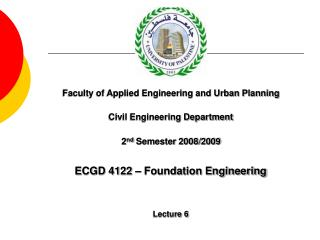DownloadDownload PresentationECGD 4122 – Foundation Engineering Lecture 6

# ECGD 4122 – Foundation Engineering Lecture 6

Download Presentation## ECGD 4122 – Foundation Engineering Lecture 6

- - - - - - - - - - - - - - - - - - - - - - - - - - - E N D - - - - - - - - - - - - - - - - - - - - - - - - - - -
##### Presentation Transcript

1. Faculty of Applied Engineering and Urban Planning Civil Engineering Department 2nd Semester 2008/2009 ECGD 4122 – Foundation Engineering Lecture 6

2. Content • Eccentrically Loaded Foundations • Foundations with two-way eccentricity • Field Load Test

3. Stress Concept

4. Stress Distribution – Rigid Footings

5. Effect of Eccentricity

6. Effect of Eccentricity Q = total vertical load on the foundation M = moment on the foundation e = M/Q

7. Effect of Eccentricity Meyerhof’s Principle Of Effective Area Method

8. Ultimate Load with Eccentricity Step 1: Find Eccentricity, If e ≤ B/6 If e > B/6

9. Ultimate Load with Eccentricity Step 2: Determine the Effective Dimensions If eccentricity is in the direction of B B΄= B – 2e L΄ = L If eccentricity is in the direction of L B΄= B L΄ = L – 2e

11. Ultimate Load with Eccentricity Step 3:Use general equation to calculate the soil ultimate bearing capacity q'u= c΄ Nc Fcs Fcd Fci + q Nq Fqs Fqd Fqi + 0.5 γ B΄Fγs Fγd Fγi USE B΄ to calculate: Fcs , Fqs , Fγs DON’T use B΄ to calculate: Fcd , Fqd , Fγd

12. Ultimate Load with Eccentricity Step 4:Determine the total ultimate load: Qult = qult A΄ = qult B΄ L΄ Step 5:Factor of safety against bearing failure:

13. Example 1 For the footing shown below, determine the ultimate vertical loading with an 0.15 m eccentricity using the general formula. Take Nq = 18.4, N = 22.4.

14. Example 1 - Solution

15. Example 1 - Solution

16. Example 1 - Solution

17. Two Way Eccentricity

18. Two Way Eccentricity

19. Two Way Eccentricity USE B΄ and L΄ to calculate: Fcs , Fqs , Fγs DON’T use B΄ and L΄ to calculate: Fcd , Fqd , Fγd

20. Two Way Eccentricity Case I: eL/L ≥ 1/6 and eB/B ≥ 1/6

21. Two Way Eccentricity Case II: eL/L < 0.5 and 0 < eB/B < 1/6

22. Two Way Eccentricity

23. Two Way Eccentricity Case III: eL/L < 1/6 and 0 < eB/B < 0.5

24. Two Way Eccentricity

25. Two Way Eccentricity Case IV: eL/L < 1/6 and eB/B < 1/6

26. Two Way Eccentricity

27. Example 2 Refer to Example 1 and the figure shown below to determine the ultimate vertical loading using the general formula.

28. Example 2 - Solution

29. Example 2 - Solution

30. Example 2 - Solution

31. Example 2 - Solution